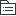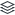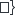python

python知识点简单总结，参考菜鸟教程，陆续更新中。。。。。

|
38

相关模板推荐

•python —— 作品大纲

•开发环境
•基础语法
• 缩进
• 标识符
• 多行连接符 \
• 引号
• 单引号，双引号
• 三引号
• 注释
• #
• 单引号 '''
• 双引号 """
• 同一行多条语句使用；隔开
• print
• print加end参数可控制结尾符
• 命令行参数
• 链式赋值
•标准数据类型
• Number
• int
• 表示长整型，没有long类型
• bool
• Ture, False
• 值是1， 0， 可与数字相加
• float
• complex
• String
• 单双引号相同
• 三引号表注释
• 转义 \
• r可以不让\转义
• +
• *
• 索引
• 从0开始
• 从-1开始
• 没有字符类型
• 截取：变量[头下标：尾下标：步长]
• Tuple
• 用()定义
• 元素不可修改
• 元组的元素可以是可变类型，如list
• 其他和列表用法一致
•不可变数据类型
• List
• 列表里元素类型可以不同
• List写在方括号之间，元素用逗号隔开
• 可以截取，方法和字符串一致
• 可以使用+拼接
• Set
• 使用大括号 { } 或者 set() 函数创建
• 空集合必须用 set()
• set会自动去除重复元素
• set可以进行集合运算
• 差集 -
• 并集 |
• 交集 &
• 异或 ^
• Dictionary
• 用 { } 标识，它是一个无序的 键(key) : 值(value) 的集合
• key
• 必须使用不可变类型
• 必须唯一
• 方法
• clear()
• keys()
• values()
•可变数据类型
•数据类型转换
• int，float，complex，str，tuple，list，set，dict
• repr(x)
• 将对象 x 转换为表达式字符串
• eval(str)
• 用来计算在字符串中的有效Python表达式,并返回一个对象
• frozenset(s)
• 转换为不可变集合
• chr(x)
• 将一个整数转换为一个字符
• ord(x)
• 将一个字符转换为它的整数值
• 进制转换
• hex(x)，oct(x)
•运算符
• 算术运算符
• + - * %
• / 结果去小数
• // 取整除，去除小数部分
• ** 幂运算
• 比较运算符
• == , != , > , < , >=, <=
• 赋值运算符
• =, +=, -=, *=, /=, %=, **=, //==
• 位运算符
• &, |, ~, ^, << , >>
• 逻辑运算符
• and
• x and y, x 为 False，返回 False，否则返回 y 的计算值
• or
• x or y, x 为 True，返回 x 的值，否则它返回 y 的计算值
• not
• x not y, x 为 True，返回 False 。 x 为 False，它返回 True
• 成员运算符
• in
• not in
• 身份运算符
• is
• 判断id是否相同
• is not
•常用内置函数
• id()
• type()
• 不会认为子类是一种父类类型
• isinstance()
• 会认为子类是一种父类类型
•函数
••编码规范
• 空行
• 函数之间，类的方法之间